Refer to our Texas Go Math Grade 5 Answer Key Pdf to score good marks in the exams. Test yourself by practicing the problems from Texas Go Math Grade 5 Lesson 11.1 Answer Key Polygons.

Essential Question
How can you identify and classify polygons?
A polygon is a two-dimensional closed figure that has three or more straight sides. Any figure with straight edges, such as a triangle or rectangle, is a polygon. Figures that have any curved sides or open sides are not classed as polygons
Polygons are classified according to their number of sides, according to their angles, and are also classified according to their equality or inequality of their angles and sides, that is, whether they are regular or irregular

Unlock the Problem
The Castel del Monte in Apulia, Italy, was built more than 750 years ago. The fortress has one central building with eight surrounding towers. Which polygon do you see repeated in the structure? How many sides, angles, and vertices does this polygon have?A polygon is a closed plane figure formed by three or more line segments that meet at points called vertices. It is named by the number of sides and angles it has. To identify the repeated polygon in the fortress, complete the tables below.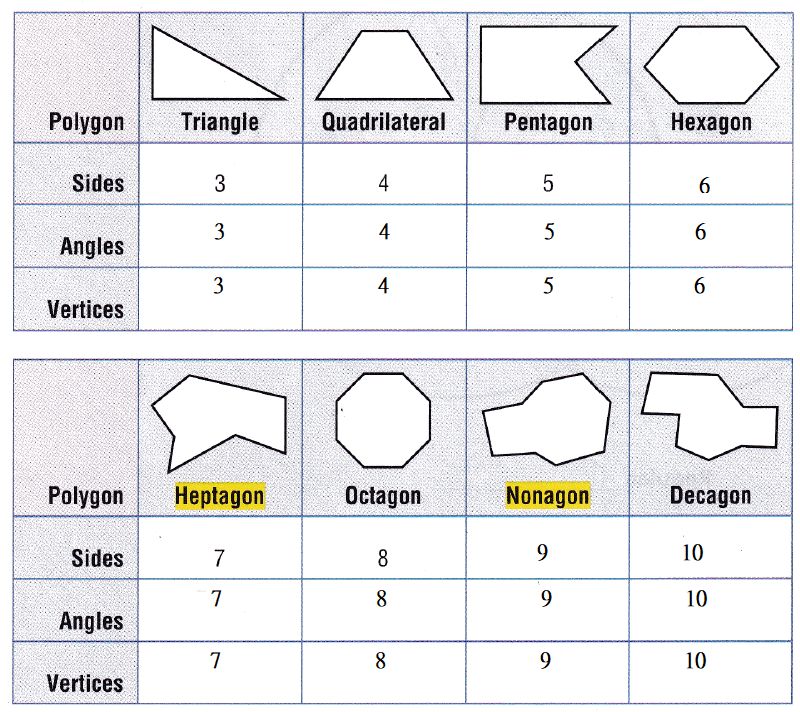So,
The “Octagon” is the repeated polygon in the Castel del Monte because
It has 8 sides, 8 angles, and 8 vertices.

Math Talk
Mathematical Processes
What pattern do you see among the number of sides, angles, and vertices a polygon has?
Based on the number of sides,
Polygons are classified into 2 types. They are:
a. Regular Polygons
b. Irregular Polygons
In Regular Polygons,
All the sides lengths and angle measures are equal
In Irregular Polygons,
The side lengths and angle measures are not equal

Try This! Label the Venn diagram to classify the polygons in each group. Then draw a polygon that belongs only to each group.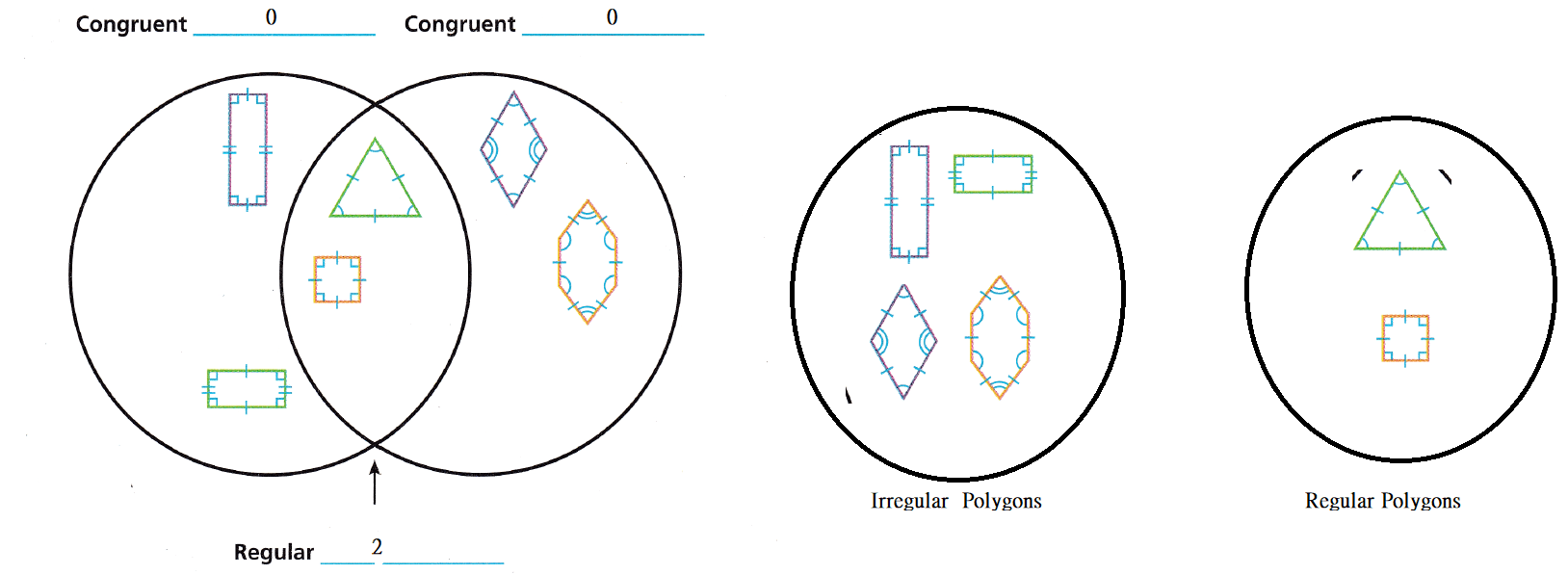Share and Show

Name each polygon. Then tell whether it is a regular polygon or not a regular polygon.

Question 1.The given polygon is:Now,
We know that,
In a Regular Polygon, the side lengths and the angle measures all must be equal
Now,
From the given figure,
We can observe that
All the side lengths are not equal
The parallel side lengths are equal
All the angle measures are equal
Hence, from the above,
We can conclude that
The given polygon is not a Regular Polygon
The name of the given Polygon is: Rectangle

Question 2.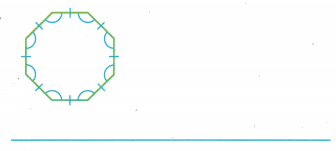The given polygon is: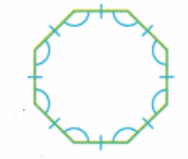Now,
We know that,
In a Regular Polygon, the side lengths and the angle measures all must be equal
Now,
From the given figure,
We can observe that
All the side lengths are equal
The parallel side lengths are equal
All the angle measures are equal
Hence, from the above,
We can conclude that
The given polygon is a Regular Polygon
The name of the given Polygon is: Octagon

Question 3.The given polygon is: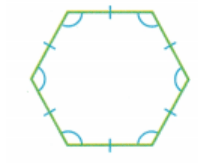Now,
We know that,
In a Regular Polygon, the side lengths and the angle measures all must be equal
Now,
From the given figure,
We can observe that
All the side lengths are equal
The parallel side lengths are equal
All the angle measures are equal
Hence, from the above,
We can conclude that
The given polygon is a Regular Polygon
The name of the given Polygon is: Hexagon

Question 4.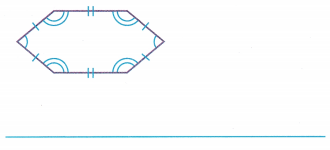The given polygon is: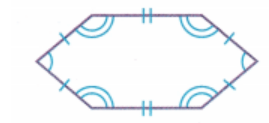Now,
We know that,
In a Regular Polygon, the side lengths and the angle measures all must be equal
Now,
From the given figure,
We can observe that
All the side lengths are not equal
The parallel side lengths are equal
All the angle measures are equal
Hence, from the above,
We can conclude that
The given polygon is not a Regular Polygon
The name of the given Polygon is: Hexagon

Problem Solving

Question 5.
H.O.T. Compare the polygons shown in Exercises 3 and 4. Use Math Language to describe how they are alike and how they are different.
The figures that will be in Exercises 3 and 4 are:Now,
From the given figures,
We can observe that
The shapes of the two figures are the same
The side lengths of the two figures are not the same
The angle measures of the two figures are not the same
One figure is a “Regular Polygon” whereas the other figure is an “Irregular Polygon”

Question 6.
Why do all regular pentagons have the same shape? Explain.
A polygon with five sides of equal length is called an equilateral pentagon. However, all the five internal angles of a pentagon can take a range of sets of values. They are thus allowing it to form a family of pentagons. Therefore, the regular pentagon is unique up to similarity.

Problem Solving

For 7-8, use the Castel del Monte floor plan at the right.

Question 7.
Multi-Step Which polygons in the floor plan have four equal sides and four congruent angles? How many of these polygons are there?The given floor plan is:Now,
From the given floor plan,
We can observe that
The polygon in the given floor plan that has four sides and four angles congruent is: Octagon
The number of Octagons in the given floor plan are: 8 Octagons

Question 8.
Multi-Step Is there a quadrilateral in the floor plan that is not a regular polygon? Name the quadrilateral and tell how many of the quadrilaterals are in the floor plan.
The given floor plan is:Now,
From the given floor plan,
We can observe that
There is a quadrilateral in the given floor plan that is not a regular polygon
The quadrilateral that is not a regular polygon in the given floor plan is: Trapezoid
The number of Trapezoids in the given floor plan are: 8

Question 9.
H.O.T. Look at the angles for all regular polygons. As the number of sides increases, do the measures of the angles increase or decrease? What pattern do you see?Question 10.
Marianna would like to create a mosaic using pieces that have only four angles. Which shapes could Marianna use?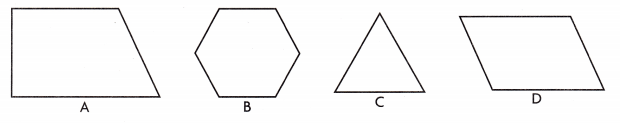(A) A and B
(B) B and C
(C) A and D
(D) B and D
It is given that
Marianna would like to create a mosaic using pieces that have only four angles
Hence, from the above,
We can conclude that
The shapes that Marianna could use is: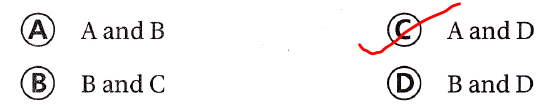Question 11.
Lino found a small green piece of glass with this shape. What is the name of the shape? Is it regular?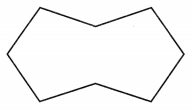(A) hexagon; regular
(B) octagon; regular
(C) hexagon; not regular
(D) octagon; not regular
It is given that
Lino found a small green piece of glass with this shape
Now,
The given small piece of glass is:Now,
From the given figure,
We can observe that
There are 8 sides in the given small piece of glass
The shape is not regular
Hence, from the above,
We can conclude that
The shape of the small piece of glass is: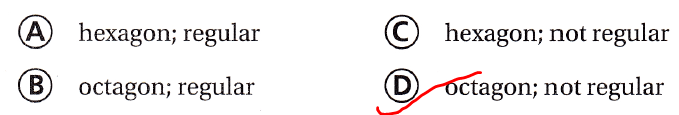Question 12.
Multi-Step Lois began a mosaic using congruent regular triangles. She first placed a triangle as a centerpiece. She then placed three triangles so that one side of each triangle lined up with one side of the original triangle. What shape was formed by the first four pieces?
(A) regular hexagon
(C) regular triangle
(D) nonagon
It is given that
Lois began a mosaic using congruent regular triangles. She first placed a triangle as a centerpiece. She then placed three triangles so that one side of each triangle lined up with one side of the original triangle
Hence, from the above,
We can conclude that
The shape that was formed by the first four pieces is:Texas Test Prep

Question 13.
Which of the following is a regular hexagon?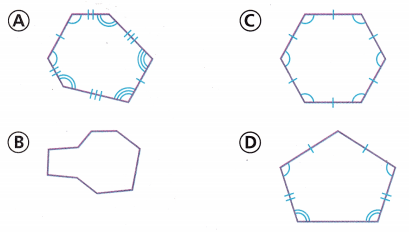We know that,
A “regular Polygon” is a polygon that has equal side lengths and equal angle measures
Hence, from the above,
We can conclude that
The regular hexagon from the following given figures is: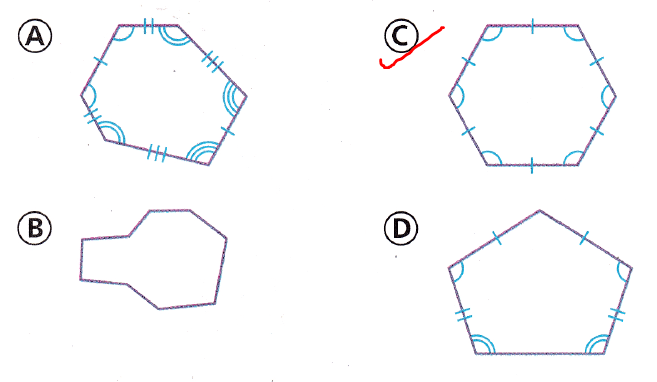### Texas Go Math Grade 5 Lesson 11.1 Homework and Practice Answer Key

Name each polygon. Then tell whether It is a regular polygon or not a regular polygon.

Question 1.The given polygon is: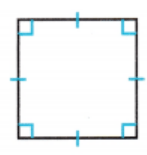Now,
We know that,
In a Regular Polygon, the side lengths and the angle measures all must be equal
Now,
From the given figure,
We can observe that
All the side lengths are equal
The parallel side lengths are equal
All the angle measures are equal
Hence, from the above,
We can conclude that
The given polygon is a Regular Polygon
The name of the given Polygon is: Square

Question 2.The given polygon is: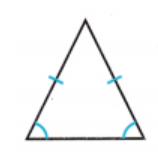Now,
We know that,
In a Regular Polygon, the side lengths and the angle measures all must be equal
Now,
From the given figure,
We can observe that
All the side lengths are equal
All the angle measures are equal
Hence, from the above,
We can conclude that
The given polygon is a Regular Polygon
The name of the given Polygon is: Triangle

Question 3.The given polygon is: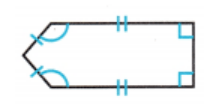Now,
We know that,
In a Regular Polygon, the side lengths and the angle measures all must be equal
Now,
From the given figure,
We can observe that
All the side lengths are not equal
The parallel sides are equal
All the angle measures are not equal
Hence, from the above,
We can conclude that
The given polygon is not a Regular Polygon
The name of the given Polygon is: Pentagon

Question 4.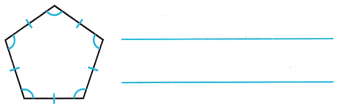The given polygon is: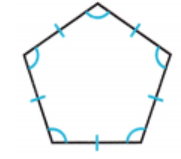Now,
We know that,
In a Regular Polygon, the side lengths and the angle measures all must be equal
Now,
From the given figure,
We can observe that
All the side lengths are equal
The parallel sides are equal
All the angle measures are equal
Hence, from the above,
We can conclude that
The given polygon is a Regular Polygon
The name of the given Polygon is: Pentagon

Problem Solving

Question 5.
Chantal draws a polygon with seven congruent sides and angles. Name Chantai’s shape. Is the shape a regular polygon? Explain.
It is given that
Chantal draws a polygon with seven congruent sides and angles
Now,
According to the given information,
The shape drawn by Chantal is: Heptagon
Since there are congruent sides and angles, “Heptagon” is a regular polygon
Hence, from the above,
We can conclude that
The shape drawn by Chantal is: Heptagon
Since there are congruent sides and angles, “Heptagon” is a regular polygon

Question 6.
Name the shapes that make up the Texas state flag. Are any of the shapes regular polygons? Explain.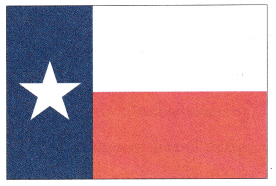The given Texas state flag is:Now,
From the given Texas state flag,
We can observe that
The flag is made up of 3 Rectangles
Now,
We know that,
In a Rectangle,
All the sides are not equal
The parallel sides are equal
All the angle measures are equal
So,
There are not any shapes that are “Regular Polygons” in the Texas state flag
Hence, from the above,
We can conclude that
There are not any shapes that are “Regular Polygons” in the Texas state flag

Texas Test Prep

Lesson Check

Question 7.
Which of the following is a regular octagon?We know that,
In a regular Octagon,
All 8 sides are equal and all the angle measures are equal
Hence, from the above,
We can conclude that
The regular Octagon from the following given figures is: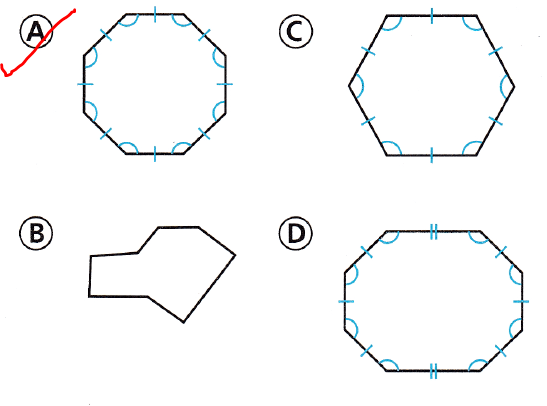Question 8.
Bradley uses only regular polygons in his shape mobile. Which polygon could NOT be in Bradley’s mobile?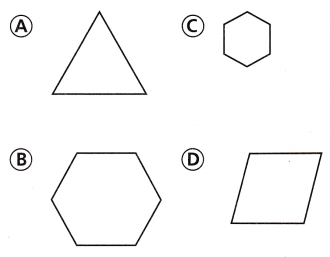It is given that
Bradley uses only regular polygons in his shape mobile
Now,
We know that,
The “Parallelogram” has the parallel equal side lengths
Hence, from the above,
We can conclude that
The shapes that could not be in Bradley’s mobile is: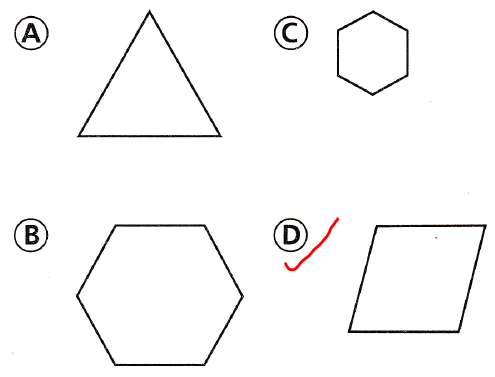Question 9.
How many regular triangles are in this figure?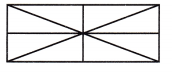(A) 0
(B) 8
(C) 4
(D) 6
The given figure is:Hence, from the above,
We can conclude that
The number of triangles in the given figure is: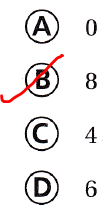Question 10.
An artist made a pendant with the shape shown below. What is the name of the shape? Is it regular?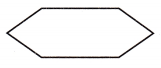(A) hexagon; regular
(B) hexagon; not regular
(C) octagon; regular
(D) octagon; not regular
It is given that
An artist made a pendant with the shape shown below
Now,
The given shape is:Now,
From the given shape,
We can observe that
There are 6 sides
All the sides and the angles are equal
Hence, from the above,
We can conclude that
The name of the given shape is:Question 11.
Multi-Step Which are the next two polygons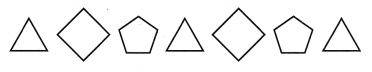(A) triangle; square
(D) pentagon; triangle
The given pattern is:Now,
From the above pattern,
We can observe that
The figures after the given pattern are: Quadrilateral, Pentagon
Hence, from the above,
We can conclude that
The next two polygons in the given pattern are: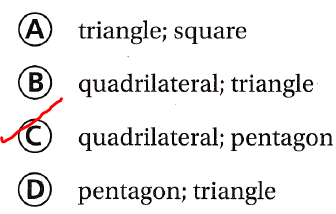Question 12.
Multi-Step How can you classify the sides in the pattern? and angles of a square?
(A) 2 pairs of congruent sides; 2 pairs of congruent angles
(B) 4 congruent angles; 4 congruent sides
(C) 0 congruent angles; 4 congruent sides
(D) 2 pairs of congruent sides; 1 pair of congruent angles